art theory 101~ Butterfly Primes

Butterfly Primes
~let the beauty seep in~

Date completed: 12-07-05

Introduction

The lure and elusiveness of prime numbers, those very same numbers which all the other natural whole numbers can be reduced to, is thousands of years in the making, captivating the minds of anyone...perhaps everyone...who has ever contemplated their beauty and mystery. Can this beauty reveal a visual and mathematical pattern?

Given Prime Definition

A prime number ( P ) is any natural whole number ( N ), greater than one, which is reducible only by itself and one.

Postulate 1: "Axis vs. Matrix, and Unique Squares"

On a Multiplication Matrix (Table) where all natural whole numbers 1,2,3,...are placed on the x- and y-axis with 1 at the origin, it follows that (Fig 1, Table I):

a. All primes, and only primes, remain when all the matrix numbers ( NM ) are eliminated from all the axial (x- or y-axis) numbers, as:

P = Nx-axis - NM                   (1)

b. Similarly, only primes remain when the product of all x-axis numbers times the y-axis numbers are eliminated from all numbers, as:

P = N-(Nx-axis x N y-axis)         (2)

c. And again, as all primes are odd (except P=2=P2 ), only primes remain when all odd matrix numbers are eliminated from all the odd numbers (plus N2=2 ), as:

P = (Nodd-x-axis + N2)-NM-odd       (3)

d. Not only are the squares of all primes (except P2 ) odd, and (except P5 ) end in either 1 or 9, the squares of the squares all end in 1, as:

P2except P2 = Nodd-ends in 1 or 9    (4)

(P2)2 = Nodd-ends in 1               (5)

e. The squares of all primes are the only unique numbers on the matrix, as:

P2 = NM-unique .                 (6)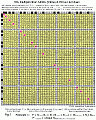Fig. 1
(click to enlarge image)
Best viewed 800x600, F-11 key

Table I

 ``` Table I. Postulate 1 All numbers, All Matrix numbers, All Prime numbers, N x-axis - NM = P 2-50... 4-50... from 1-50... as a group as a group as a group ____________ ___________________ __________________ - 4 2 6 2 3 8 3 4 9 5 10 5 6 12 7 14 7 8 15 9 16 10 18 11 20 11 12 21 13 22 13 14 24 15 25 16 26 17 27 17 18 28 19 30 19 20 32 21 33 22 34 23 35 23 24 36 25 38 26 39 27 40 28 42 29 44 29 30 45 31 46 31 32 48 33 49 34 50 35 36 37 37 38 39 40 41 41 42 43 44 45 46 47 47 48 49 50 ```

Commentary

All natural whole numbers, N = 1, 2, 3,... , placed on the x- and y-axis, with the unit number 1 at the origin to form the Multiplication Matrix (Table). The Multiplication Matrix Numbers, NM , equals the grid of products formed from multiplying the Nx-axis and Ny-axis (excluding N=1 ) numbers. All prime numbers, P , are only found, by definition, on the x- or y-axis and are not part of the matrix numbers, NM . Removing (not actually subtracting) all the numbers from this axis which also appear in the matrix table will leave only the prime numbers. The corollary to this is that the squares of the primes are the only unique numbers within the matrix table. Fig.1.

Postulate 2: "Divisible Differences Predict Primes"

a. Except for P2,3,5 the difference in the squares of any prime is divisible by 24, as (Fig.2, Table II):

(P>)2 - (P<)2 = n24                (7)

[(P>)2 - (P<)2] / 24 = n           (8)

[(P>)2 - (P<)2] / n = 24     v      (9)

where P> = greater, P7 or higher, and, P< = lesser

b. The square root of any squared prime plus a multiple of 24 (n24) equals the next prime, as:

Pnext = [(P<)2 + n24]½             (10)

where P< = P5 or higher.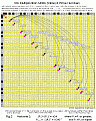Fig. 2
(click to enlarge image)
Best viewed 800x600, F-11 key

Table II

 ``` Table II Postulate 2 All (a.) (b.) Primes, | P squared (P>)2 - (P<)2 = n24 | n24+(P<)2 = P2next (P2next)1/2 = P ____ ______________ _____ | __________ _______ __________ _____ 2 | 3 | 5 ^2 = 25 25 -9 = | 7 ^2 = 49 49 -25 = (1)24 | (1)24 + 25 = 49 49 7 11 ^2 = 121 121 -49 = (3)24 | (3)24 + 49 = 121 121 11 13 ^2 = 169 169 -121 = (2)24 | (2)24 + 121 = 169 169 13 17 ^2 = 289 289 -169 = (5)24 | (5)24 + 169 = 289 289 17 19 ^2 = 361 361 -289 = (3)24 | (3)24 + 289 = 361 361 19 23 ^2 = 529 529 -361 = (7)24 | (7)24 + 361 = 529 529 23 29 ^2 = 841 841 -529 = (13)24 | (13)24 + 529 = 841 841 29 31 ^2 = 961 961 -841 = (5)24 | (5)24 + 841 = 961 961 31 37 ^2 = 1369 1369-961 = (17)24 | (17)24 + 961 = 1369 1369 37 41 ^2 = 1681 1681-1369= (13)24 | (13)24 + 1369 = 1681 1681 41 43 ^2 = 1849 1849-1681= (7)24 | (7)24 + 1681 = 1849 1849 43 47 ^2 = 2209 2209-1849= (15)24 | (15)24 + 1849 = 2209 2209 47 ~~~~~~~~~~~~~~~~~~~~~~~~~~~~~~~~~~~~~~~~~~~~~~~~~~~~~~~~~~~~~~~~~~~~~~~~~~~~~~~~~~~~~~ 53 ^2 = 2809 2809-2209= (25)24 | (25)24 + 2209 = 2809 2809 53 59 ^2 = 3481 3481-2809= (28)24 | (28)24 + 2809 = 3481 3481 59 61 ^2 = 3721 3721-3481= (10)24 | (10)24 + 3481 = 3721 3721 61 67 ^2 = 4489 4489-3721= (32)24 | (32)24 + 3721 = 4489 4489 67 71 ^2 = 5041 5041-4489= (23)24 | (23)24 + 4489 = 5041 5041 71 73 ^2 = 5329 5329-5041= (12)24 | (12)24 + 5041 = 5329 5329 73 79 ^2 = 6241 6241-5329= (38)24 | (38)24 + 5329 = 6241 6241 79 83 ^2 = 6889 6889-6241= (27)24 | (27)24 + 6241 = 6889 6889 83 89 ^2 = 7921 7921-6889= (43)24 | (43)24 + 6889 = 7921 7921 89 97 ^2 = 9409 9409-7921= (62)24 | (62)24 + 7921 = 9409 9409 97 ~~~~~~~~~~~~~~~~~~~~~~~~~~~~~~~~~~~~~~~~~~~~~~~~~~~~~~~~~~~~~~~~~~~~~~~~~~~~~~~~~~~~~~~ 101 ^2 = 10201 10201-9409= (33)24 | (33)24 + 9409 = 10201 10201 101 103 ^2 = 10609 10609-10201= (17)24 | (17)24 +10201 = 10609 10609 103 107 ^2 = 11449 11449-10609= (35)24 | (35)24 +10609 = 11449 11449 107 109 ^2 = 11881 11881-11449= (18)24 | (18)24 +11449 = 11881 11881 109 113 ^2 = 12769 12769-11881= (37)24 | (37)24 +11881 = 12769 12769 113 127 ^2 = 16129 16129-12769= (140)24 |(140)24 +12769 = 16129 16129 127 131 ^2 = 17161 17161-16129= (43)24 | (43)24 +16129 = 17161 17161 131 137 ^2 = 18769 18769-17161= (67)24 | (67)24 +17161 = 18769 18769 137 139 ^2 = 19321 19321-18769= (23)24 | (23)24 +18769 = 19321 19321 139 149 ^2 = 22201 22201-19321= (120)24 |(120)24 +19321 = 22201 22201 149 ~~~~~~~~~~~~~~~~~~~~~~~~~~~~~~~~~~~~~~~~~~~~~~~~~~~~~~~~~~~~~~~~~~~~~~~~~~~~~~~~~~~~~~~ 151 ^2 = 22801 22801-22201= (25)24 | (25)24 +22201 = 22801 22801 151 157 ^2 = 24649 24649-22801= (77)24 | (77)24 +22801 = 24649 24649 157 163 ^2 = 26569 26569-24649= (80)24 | (80)24 +24649 = 26569 26569 163 167 ^2 = 27889 27889-26569= (55)24 | (55)24 +26569 = 27889 27889 167 173 ^2 = 29929 29929-27889= (85)24 | (85)24 +27889 = 29929 29929 173 179 ^2 = 32041 32041-29929= (88)24 | (88)24 +29929 = 32041 32041 179 181 ^2 = 32761 32761-32041= (30)24 | (30)24 +32041 = 32761 32761 181 191 ^2 = 36481 36481-32761= (155)24 |(155)24 +32761 = 36481 36481 191 193 ^2 = 37249 37249-36481= (32)24 | (32)24 +36481 = 37249 37249 193 197 ^2 = 38809 38809-37249= (65)24 | (65)24 +37249 = 38809 38809 197 199 ^2 = 39601 39601-38809= (33)24 | (33)24 +38809 = 39601 39601 199 ~~~~~~~~~~~~~~~~~~~~~~~~~~~~~~~~~~~~~~~~~~~~~~~~~~~~~~~~~~~~~~~~~~~~~~~~~~~~~~~~~~~~~~~ 211 ^2 = 44521 44521-39601= (205)24 |(205)24 +39601 = 44521 44521 211 223 ^2 = 49729 49729-44521= (217)24 |(217)24 +44521 = 49729 49729 223 227 ^2 = 51529 51529-49729= (75)24 | (75)24 +49729 = 51529 51529 227 ~~~~~~~~~random clusters hereafter through the first 10K primes~~~~~~~~~~~ ~~~~~~~~~~~~~~~~~~~~~~~~~~~~~~~~~~~~~~~~~~~~~~~~~~~~~~~~~~~~~~~~~~~~~~~~~~~~~~~~~~~~~~~ 283 ^2 = 80089 | 293 ^2 = 85849 85849-80089= (240)24 |(240)24 + 80089 = 85849 85849 293 307 ^2 = 94249 94249-85849= (350)24 |(350)24 + 85849 = 94249 94249 307 | 449 ^2 = 201601 | 457 ^2 = 208849 208849-201601=(302)24 |(302)24 +201601 = 208849 208849 457 461 ^2 = 212521 212521-208849=(153)24 |(153)24 +208849 = 212521 212521 461 | 617 ^2 = 380689 | 619 ^2 = 383161 383161-380689=(103)24 |(103)24 +380689 = 383161 383161 619 631 ^2 = 398161 398161-383161=(625)24 |(625)24 +383161 = 398161 398161 631 | 787 ^2 = 619369 | 797 ^2 = 635209 635209-619369=(660)24 |(660)24 +619369 = 635209 635209 797 809 ^2 = 654481 654481-635209=(803)24 |(803)24 +635209 = 654481 654481 809 ~~~~~~~~~~~~~~~~~~~~~~~~~~~~~~~~~~~~~~~~~~~~~~~~~~~~~~~~~~~~~~~~~~~~~~~~~~~~~~~~~~~~~~~~ 2729 ^2 = 7447441 | 2731 ^2 = 7458361 7458361-7447441= (455)24 | (455)24 +7447441 = 7458361 7458361 2731 2741 ^2 = 7513081 7513081-7458361=(2280)24 |(2280)24 +7458361 = 7513081 7513081 2741 ~~~~~~~ headings combined to save space~~~~~~~~~~~~~ 4691 ^2 = 22005481 4703 ^2 = 22118209 -22005481= (4697)24 | (4697)24 +22005481 = 22118209 ^1/2 4703 4721 ^2 = 22287841 -22118209= (7068)24 | (7068)24 +22118209 = 22287841 ^1/2 4721 5749 ^2 = 33051001 5779 ^2 = 33396841 -33051001= (14410)24 | (14410)24 +33051001 = 33396841 ^1/2 5779 5783 ^2 = 33443089 -33396841= (1927)24 | (1927)24 +33396841 = 33443089 ^1/2 5783 6841 ^2 = 46799281 6857 ^2 = 47018449 -46799281= (9132)24 | (9132)24 +46799281 = 47018449 ^1/2 6857 6863 ^2 = 47100769 -47018449= (3430)24 | (3430)24 +47018449 = 47100769 ^1/2 6863 7901 ^2 = 62425801 7907 ^2 = 62520649 -62425801= (3952)24 | (3952)24 +62425801 = 62520649 ^1/2 7907 7919 ^2 = 62710561 -62520649= (7913)24 | (7913)24 +62520649 = 62710561 ^1/2 7919 8231 ^2 = 67749361 8233 ^2 = 67782289 -67749361= (1372)24 | (1372)24 +67749361 = 67782289 ^1/2 8233 8237 ^2 = 67848169 -67782289= (2745)24 | (2745)24 +67782289 = 67848169 ^1/2 8237 9719 ^2 = 94458961 9721 ^2 = 94497841 -94458961= (1620)24 | (1620)24 +94458961 = 94497841 ^1/2 9721 9733 ^2 = 94731289 -94497841= (9727)24 | (9727)24 +94497841 = 94731289 ^1/2 9733 ~~~~~~~~~~~~~~~~~~~~~~~~~~~~~~~~~~~~~~~~~~~~~~~~~~~~~~~~~~~~~~~~~~~~~~~~~~~~~~~~~~~~~~~~~~ 10141 ^2 = 102839881 10151 ^2 = 103042801 -102839881= (8455)24 | (8455)24 +102839881 =103042801 ^1/2 10151 10159 ^2 = 103205281 -103042801= (6770)24 | (6770)24 +103042801 =103205281 ^1/2 10159 11003 ^2 = 121066009 11027 ^2 = 121594729-121066009= (22030)24 | (22030)24 +121066009 =121594729 ^1/2 11027 11047 ^2 = 122036209-121594729= (18395)24 | (18395)24 +121594729 =122036209 ^1/2 11047 12941 ^2 = 167469481 12953 ^2 = 167780209-167469481= (12947)24 | (12947)24 +167469481 =167780209 ^1/2 12953 12959 ^2 = 167935681-167780209= (6478)24 | (6478)24 +167780209 =167935681 ^1/2 12959 13033 ^2 = 169859089 13037 ^2 = 169963369-169859089= (4345)24 | (4345)24 +169859089 =169963369 ^1/2 13037 13043 ^2 = 170119849-169963369= (6520)24 | (6520)24 +169963369 =170119849 ^1/2 13043 14407 ^2 = 207561649 14411 ^2 = 207676921-207561649= (4803)24 | (4803)24 +207561649 =207676921 ^1/2 14411 14419 ^2 = 207907561-207676921= (9610)24 | (9610)24 +207676921 =207907561 ^1/2 14419 15937 ^2 = 253987969 15959 ^2 = 254689681-253987969= (29238)24 | (29238)24 +253987969 =254689681 ^1/2 15959 15971 ^2 = 255072841-254689681= (15965)24 | (15965)24 +254689681 =255072841 ^1/2 15971 17851 ^2 = 318658201 17863 ^2 = 319086769-318658201= (17857)24 | (17857)24 +318658201 =319086769 ^1/2 17863 17881 ^2 = 319730161-319086769= (26808)24 | (26808)24 +319086769 =319730161 ^1/2 17881 19543 ^2 = 381928849 19553 ^2 = 382319809-381928849= (16290)24 | (16290)24 +381928849 =382319809 ^1/2 19553 19559 ^2 = 382554481-382319809= (9778)24 | (9778)24 +382319809 =382554481 ^1/2 19559 27739 ^2 = 769452121 27743 ^2 = 769674049-769452121= (9247)24 | (9247)24 +769452121 =769674049 ^1/2 27743 27749 ^2 = 770007001-769674049= (13873)24 | (13873)24 +769674049 =770007001 ^1/2 27749 37097 ^2 = 1376187409 37117 ^2 = 1377671689-1376187409=(61845)24 | (61845)24 +1376187409 =1377671689 ^1/2 37117 37123 ^2 = 1378117129-1377671689=(18560)24 | (18560)24 +1377671689 =1378117129 ^1/2 37123 46687 ^2 = 2179675969 46691 ^2 = 2180049481-2179675969= (15563)24 | (15563)24 +2179675969 =2180049481 ^1/2 46691 46703 ^2 = 2181170209-2180049481= (46697)24 | (46697)24 +2180049481 =2181170209 ^1/2 46703 50123 ^2 = 2512315129 50129 ^2 = 2512916641-2512315129= (25063)24 | (25063)24 +2512315129 =2512916641 ^1/2 50129 50131 ^2 = 2513117161-2512916641= (8355)24 | (8355)24 +2512916641 =2513117161 ^1/2 50131 60773 ^2 = 3693357529 60779 ^2 = 3694086841-3693357529= (30388)24 | (30388)24 +3693357529 =3694086841 ^1/2 60779 60793 ^2 = 3695788849-3694086841= (70917)24 | (70917)24 +3694086841 =3695788849 ^1/2 60793 79867 ^2 = 6378737689 79873 ^2 = 6379696129-6378737689= (39935)24 | (39935)24 +6378737689 =6379696129 ^1/2 79873 79889 ^2 = 6382252321-6379696129=(106508)24 |(106508)24 +6379696129 =6382252321 ^1/2 79889 82963 ^2 = 6882859369 82981 ^2 = 6885846361-6882859369=(124458)24 |(124458)24 +6882859369 =6885846361 ^1/2 82981 82997 ^2 = 6888502009-6885846361=(110652)24 |(110652)24 +6885846361 =6888502009 ^1/2 82997 92461 ^2 = 8549036521 92467 ^2 = 8550146089-8549036521= (46232)24 | (46232)24 + 8549036521 =8550146089 ^1/2 92467 92479 ^2 = 8552365441-8550146089= (92473)24 | (92473)24 + 8550146089 =8552365441 ^1/2 92479 99989 ^2= 9997800121 99991 ^2= 9998200081-9997800121= (16665)24 | (16665)24 +9997800121 =9998200081 ^1/2 99991 100003 ^2=10000600009-9998200081= (99997)24 | (99997)24 +9998200081 =10000600009 ^1/2 100003 100019 ^2=10003800361-10000600009=(133348)24|(133348)24 +10000600009=10003800361 ^1/2 100019 104309 ^2=10880367481 104311 ^2=10880784721-10880367481=(17385)24 | (17385)24 +10880367481=10880784721 ^1/2 104311 104323 ^2=10883288329-10880784721=(104317)24|(104317)24 +10880784721=10883288329 ^1/2 104323 104717 ^2=10965650089 104723 ^2=10966906729-10965650089=(52360)24 | (52360)24 +10965650089=10966906729 ^1/2 104723 104729 ^2=10968163441-10966906729=(52363)24 | (52363)24 +10966906729=10968163441 ^1/2 104729 ~~~ ```

Commentary

Take the squares of any prime number 5 or more, and the difference between it and any other squared prime number is always an exact multiple of 24, n24. Twenty-four, or a multiple of 24, when added to the square of any prime number, and the square root of that sum taken, will always equal the next prime number to follow. This follows from the smallest primes ( P5 or higher) to predicting and calculating the next largest known prime. Please donate your prize money to help fight world hunger, disease and the deterioration of the planet and/or for the education of that under-privileged child. Fig.2.

Postulate 3: "Butterfly Primes"

a. An overlap of all multiples of 24 (n24) on the Multiplication Matrix (Table) reveals a visual and mathematically logical pattern ("Butterfly"). The squared primes, five and up, are always positioned exactly between two symmetrically placed n24s along side the diagonal "squares" line...effectively selecting out all prime candidates such that, taking the square root of, all even numbers along one axis times the same numbers increased by 2 on the other axis (whose product must equal n24)...and adding one to that product, (Figs 3a, b and c, Table III) generates the candidates, as:

P2candidate = [Neven x (N+2)] + 1      (11)

Pcandidate = ([Neven x (N+2)] + 1)½    (12)

b. Applying the principles of Postulates 1 and 2 above eliminates all the spurious entries leaving only the squared primes.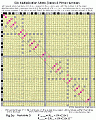Fig. 3a
(click to enlarge image)
Best viewed 800x600, F-11 key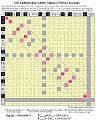Fig. 3b
(click to enlarge image)
Best viewed 800x600, F-11 key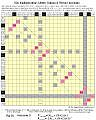Fig. 3c
(click to enlarge image)
Best viewed 800x600, F-11 key

Table III

 ``` Table III Postulate 3 [ Product must equal n24] [Neven x (N+2)] + 1 = P2candidate (P2candidate)1/2 = Pcandidate ________________________________ ____________ ______________ __________ - - >=non-prime - 4 6 1 25 25 5 6 8 1 49 49 7 10 12 1 121 121 11 12 14 1 169 169 13 16 18 1 289 289 17 ~~~~~~~~~~~~~~~~~~~~~~~~~~~~~~~~~~~~~~~~~~~~~~~~~~~~~~~~~~~~~~~~~~~~~~~~~~~ 18 20 1 361 361 19 22 24 1 529 529 23 24 26 1 625 625 >25 28 30 1 841 841 29 30 32 1 961 961 31 ~~~~~~~~~~~~~~~~~~~~~~~~~~~~~~~~~~~~~~~~~~~~~~~~~~~~~~~~~~~~~~~~~~~~~~~~~~~~ 34 36 1 1225 1225 >35 36 38 1 1369 1369 37 40 42 1 1681 1681 41 42 44 1 1849 1849 43 46 48 1 2209 2209 47 ~~~~~~~~~~~~~~~~~~~~~~~~~~~~~~~~~~~~~~~~~~~~~~~~~~~~~~~~~~~~~~~~~~~~~~~~~~~~~ 48 50 1 2401 2401 >49 52 54 1 2809 2809 53 54 56 1 3035 3035 >55 58 60 1 3481 3481 59 60 62 1 3721 3721 61 ~~~~~~~~~~~~~~~~~~~~~~~~~~~~~~~~~~~~~~~~~~~~~~~~~~~~~~~~~~~~~~~~~~~~~~~~~~~~~ 64 66 1 4225 4225 >65 66 68 1 4489 4489 67 70 72 1 5041 5041 71 72 74 1 5329 5329 73 76 78 1 5929 5929 >77 ~~~~~~~~~~~~~~~~~~~~~~~~~~~~~~~~~~~~~~~~~~~~~~~~~~~~~~~~~~~~~~~~~~~~~~~~~~~~~ 78 80 1 6241 6241 79 82 84 1 6889 6889 83 84 86 1 7225 7225 >85 88 90 1 7921 7921 89 90 92 1 8281 8281 >91 ~~~~~~~~~~~~~~~~~~~~~~~~~~~~~~~~~~~~~~~~~~~~~~~~~~~~~~~~~~~~~~~~~~~~~~~~~~~~~ 94 96 1 9025 9025 >95 96 98 1 9409 9409 97 100 102 1 10201 10201 101 102 104 1 10609 10609 103 106 108 1 11449 11449 107 ~~~~~~~~~~~~~~~~~~~~~~~~~~~~~~~~~~~~~~~~~~~~~~~~~~~~~~~~~~~~~~~~~~~~~~~~~~~~~ 108 110 1 11881 11881 109 112 114 1 12769 12769 113 114 116 1 13225 13225 >115 118 120 1 14161 14161 >119 120 122 1 14641 14641 >121 ~~~~~~~~~~~~~~~~~~~~~~~~~~~~~~~~~~~~~~~~~~~~~~~~~~~~~~~~~~~~~~~~~~~~~~~~~~~~~ 124 126 1 15625 15625 >125 126 128 1 16129 16129 127 130 132 1 17161 17161 131 132 134 1 17689 17689 >133 136 138 1 18769 18769 137 ~~~~~~~~~~~~~~~~~~~~~~~~~~~~~~~~~~~~~~~~~~~~~~~~~~~~~~~~~~~~~~~~~~~~~~~~~~~~~ ~~~~~~~~~~~~~~random samples of know primes~~~~~~~~~~~~~~~~~~~~~ 786 788 1 619369 619369 787 796 798 1 635209 635209 797 808 810 1 654481 654481 809 810 812 1 657721 657721 811 2728 2730 1 7447441 7447441 2729 2730 2732 1 7458361 7458361 2731 2740 2742 1 7513081 7513081 2741 4690 4692 1 22005481 22005481 4691 4702 4704 1 22118209 22118209 4703 4720 4722 1 22287841 22287841 4721 5748 5750 1 33051001 33051001 5749 5778 5780 1 33396841 33396841 5779 5782 5784 1 33443089 33443089 5783 6840 6842 1 46799281 46799281 6841 6856 6858 1 47018449 47018449 6857 6862 6864 1 47100769 47100769 6863 7900 7902 1 62425801 62425801 7901 7906 7908 1 62520649 62520649 7907 7918 7920 1 62710561 62710561 7919```

Commentary

Within the Multiplication Matrix (Table) there are any number of patterns found by delineating the occurrences of a particular number multiple. Here, the concern is with the number 24.

A basic, modular repeating visual pattern is found by highlighting all instances of n24 within the matrix numbers formed from the products of the 1-24 x- and y-axis numbers. This pattern is mirrored in the other three quadrants of an expanded 48 x 48 matrix, and so on...very much a fractal pattern encompassing the products of all numbers.

The original 24 x 24 fractal base pattern is itself composed of four quadrants formed by the crossings of n24s at the product lines of the 12 x- and y-axis. Because the squares of the primes all fall symmetrically on the diagonal drawn from the origin, the focus will be solely on those two quadrants hereafter...though it is to be remembered that the n24 pattern is mirrored in all quadrants as a pattern of eight internal n24s highlights. What remains in this simplification is the "Butterfly Primes" pattern...two diagonally symmetrical quadrant patterns whose eight internal, and 10 axial, n24s highlights radiate out from the center (12 x 12 =144 in this module). This pattern extends diagonally to infinity. In between each and every n24 pair which lies along side the strict diagonal "squares" line (always off the diagonal number by 1), there exists a squared prime number candidate. The pattern is of n24s shepherding the squared primes along the diagonal processional. This pattern defines and predicts all primes when the spurious candidates are eliminated by applying the principles of Postulates 1 and 2.

Twin primes ( P, P+2 ), Prime Triples ( P, P+2, P+6 or P, P+4, P+6 ) and Prime Quadruples ( P, P+2, P+6, P+8 or P, P+2, P+4, P+8 ) and other prime patterns can be seen to result from the logical beauty and order of the "Butterfly" pattern. Fig.3.

Conjecture: "Proof of Riemann's Hypothesis"

Taken together, these three postulates prove the order within the prime numbers necessary to establish a non-variance factor (n24)...the landscape at sea level (Riemann's zero points of the zeta function)...the critical line...and it is not "noisy", random or fluctuating, but constant, predictable and straight...and thus proves Riemann's Hypothesis. Each and every prime number square is shepherded along the "squares" diagonal by a series of constant, ordered and symmetrical n24s confirming the larger order of the primes within this "Butterfly" of n24s.

New Prime Definition = new "Prime Number Theorem"

A prime number is any natural whole number, greater than one, which is reducible only by itself and one, and, is always separated from the square of all other primes (except P2,3,5 ) by multiplies of 24. The infinity of primes can not exceed the infinity of n24.

Conjecture: Primes and the Inverse Square Law

The Inverse Square Law, 1/r2, so fundamental to our physical world...witness gravity and electromagnetism, light and sound, energy, etc...is built on the "odd number summation series", that is, as r, the radius (or distance) increases as 1,2,3,4,5,... the sequential difference between the squares, as r2, follows as 3,5,7,9,11,.... As the distance, r, is doubled, the influence diminishes by 1/4. When r is tripled the influence is reduced by 1/9, when r is quadrupled, the influence drops to 1/16 of the original, and so on. The odd numbers: 1...3...5...7...9... and so on, when added to each other form the sums: 1...4...9...16...25... and so on, forming the "odd number summation series"...the square roots of which form the series: 1,2,3,4,5 and so on.

The "odd number summation series can easily be seen as the diagonal "squares" line on any of the above figures. The odd numbers which form it (when squared), are made of prime and non-prime numbers. The non-prime numbers can be shown to be made of the product of two or more prime numbers. Table IV.

It follows that all odd natural whole numbers, greater than one, are either prime or composed of the product of two or more prime numbers. See the (1) Caldwell and (4) Brooks reference links below.

Table IV

 ``` Table IV. Primes and the Inverse Square Law All odd (Nodd)2 Non-Prime numbers, generates Nodd(x-axis) Nodd(x-axis) the odd numbers are 3-100..., Inverse the product form the Square of two or more difference Numbers Prime numbers, P between the Inverse Square Number's "odd number summation series" ______ _______ __________________ 3 squared = 9 5 squared = 25 7 squared = 49 9 squared = 81 9 = 3x3 11 squared = 121 13 squared = 169 15 squared = 225 15 = 3x5 17 squared = 289 19 squared = 361 21 squared = 441 21 = 3x7 23 squared = 529 25 squared = 625 25 = 5x5 27 squared = 729 27 = 3x3x3 29 squared = 841 31 squared = 961 33 squared = 1089 33 = 3x11 35 squared = 1225 35 = 5x7 37 squared = 1369 39 squared = 1521 39 = 3x13 41 squared = 1681 43 squared = 1849 45 squared = 2025 45 = 3x3x5 47 squared = 2209 49 squared = 2401 49 = 7x7 51 squared = 2601 51 = 3x17 53 squared = 2809 55 squared = 3025 55 = 5x11 57 squared = 3249 57 = 3x19 59 squared = 3481 61 squared = 3721 63 squared = 3969 63 = 3x3x7 65 squared = 4225 65 = 5x13 67 squared = 4489 69 squared = 4761 69 = 3x23 71 squared = 5041 73 squared = 5329 75 squared = 5625 75 = 3x5x5 77 squared = 5929 77 = 7x11 79 squared = 6241 81 squared = 6561 81 = 3x3x3x3 83 squared = 6889 85 squared = 7225 85 = 5x17 87 squared = 7569 87 = 3x29 89 squared = 7921 91 squared = 8281 91 = 7x13 93 squared = 8649 93 = 3x31 95 squared = 9025 95 = 5x19 97 squared = 9409 99 squared = 9801 99 = 3x3x11 and so on```

Conclusion

By using an expanded Multiplication Matrix (Table) and plotting the patterns of the prime numbers on the axis and their squares in the matrix, a strict order based on a factor of 24 was found which is both numerically and visually logical. The difference in squared primes of multiples of 24 allows for the validation of all primes past and the prediction and calculation of all new primes to be easily accomplished. Three Postulates and a new Prime Number Theorem have been offered to summarize and codify these findings...and taken together they define a beautiful ordered pattern within the primes revealing the proof of Riemann's Hypothesis.

References

The three excellent website references below provide outstanding information, presentation and resources about primes.

1. Caldwell, Chris, The Prime Pages,
http://primes.utm.edu

First and last source to check out everything you ever wanted to know about primes-history, glossary, proofs, types, lists, resources and more. Well referenced and up to date.

2. du Sautoy, Marcus, The Music of the Primes,
http://www.musicoftheprimes.com

A beautiful site which educates you as you go along the melody of mathematical thought. Particularly insightful presentation of imaginary numbers and the musical landscape metaphor elucidating Riemann's pursuit of the great ordered pattern of the primes.

3. Watkins, Matthew R., Number Theory and Physics,
http://www.maths.ex.ac.uk/~mwatkins/zeta/physics.htm

Embedded in the bigger picture of number theory and its relationship to physics, this wonderful site both teaches and inspires by relating the history of numerical and physical thought by their authors to a contemporary presentation of those ideas. Full of resources and great quotes.

Additional writings on art, math and physics by the author can be found at:

4. Brooks, Reginald, Art Theory 101,
http://www.brooksdesign-ps.net/Code/Html/arthry5.com

5. Alfeld, Peter, http://www.math.utah.edu/~alfeld/

6. Chamness, Mark, http://alumnus.caltech.edu/~chamness/Prime.html

7. Edgington, Will, http://www.garlic.com/~wedgingt/mersenne.html

8. Heinz, Harvey, http://www.geocities.com/~harveyh/primes.htm

10. The Mathematical Association of America, http://www.maa.org

11. O'Connor, John and Edmund Robertson, http://www-history.mcs.st-and.ac.uk/history/HistTopics/Prime_numbers.html

12. Peterson, Ivars, http://www.sciencenews.org

13. Woltman, George, http://www.mersenne.org/prime.htm (GIMPS)

_________________________________________________________________________________________

Use

This paper and all its contents © 2005-06, Reginald Brooks. All rights reserved. This work and all its content are, to the best knowledge of its author, original and solely of and by the author at the time of its creation. Permission is hereby granted for single copies to be made for personal, non-commercial use for students and teachers of schools, colleges and universities provided that: either the entire paper, including figures and tables, is kept intact; or, any extracts of the text, or figures or tables (in part or whole), be properly and visibly cited as to authorship and source.

Special thanks to Calc98 (http://www.calculator.org/), whose software calculator eloquently handled the big digits after running out of space on my trusty hand-held Texas Instruments Slimline TI-35 Solar Scientific Calculator

Go to Butterfly Prime Directive (white paper), or
The Butterfly Prime Determinate Number Array (white paper), or
Butterfly Primes ~Prejudicial Numbers~ (new media net.art)